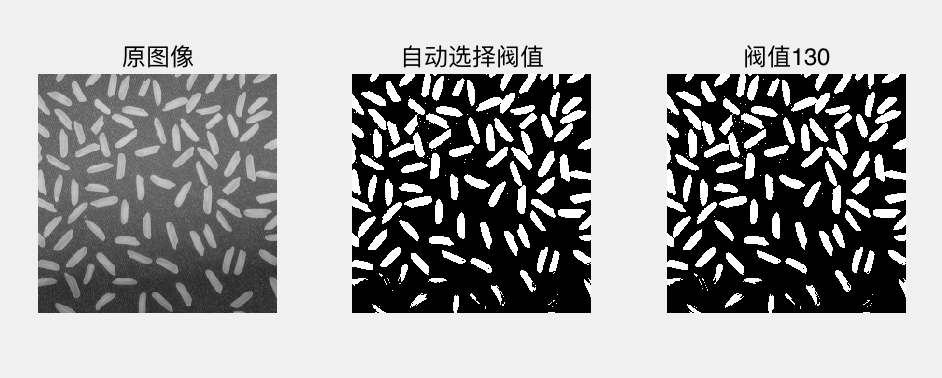# 图像的点运算

Posted by jjx on December 20, 2016

$s=T(r)$

• 最基本的图像分析工具:灰度直方图
• 利用直方图辅助实现的各种灰度变换， 包括灰度线性变换、伽马变换、阀值变换和窗 口变换等

#### 灰度直方图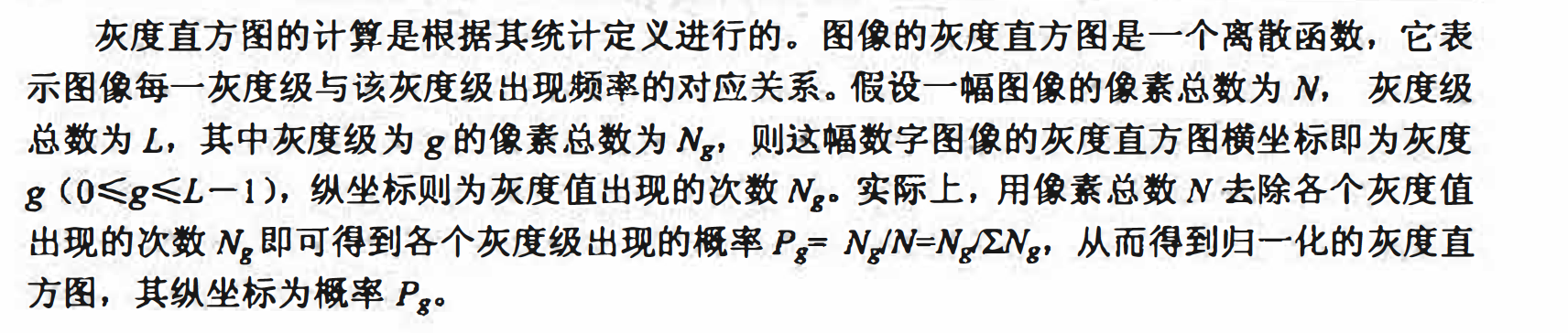matlab实现
Matlab中的imhist函数可以进行图像的灰度直方圈运算，调用语法为：

imhist(I)
imhist(I,n)
[count,x]=imhist(...)

• I为需要计算灰度直方图的图像．
• n为指定的灰度级数目． 如果指定参数n，则会将所有的灰度级均匀分布在n 个小区 间内，而不是将所有的灰度级全部分开．
• counts为直方图数据向量. counts(i）表示第i个灰度区间中的像素数目． • x是保存了对应的灰度小区间的向量．

I = imread('pout.tif');
figure;
imshow(I); title('Souce');
figure;
imhist(I);title('Graph');
figure;
imhist(I,64);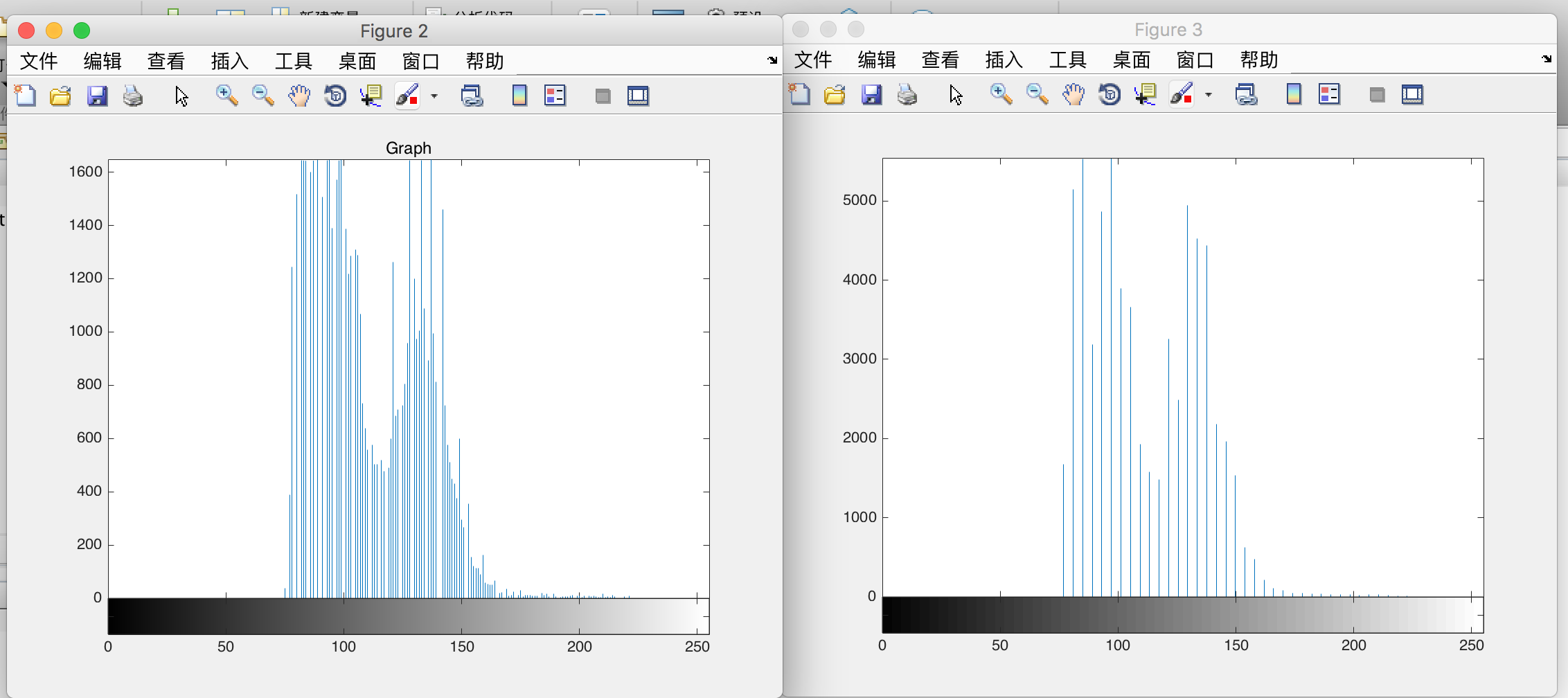I = imread('pout.tif');
figure;
[M,N]=size(I);
[counts,x]=imhist(I,32);
counts= counts / M/N;
stem(x,counts);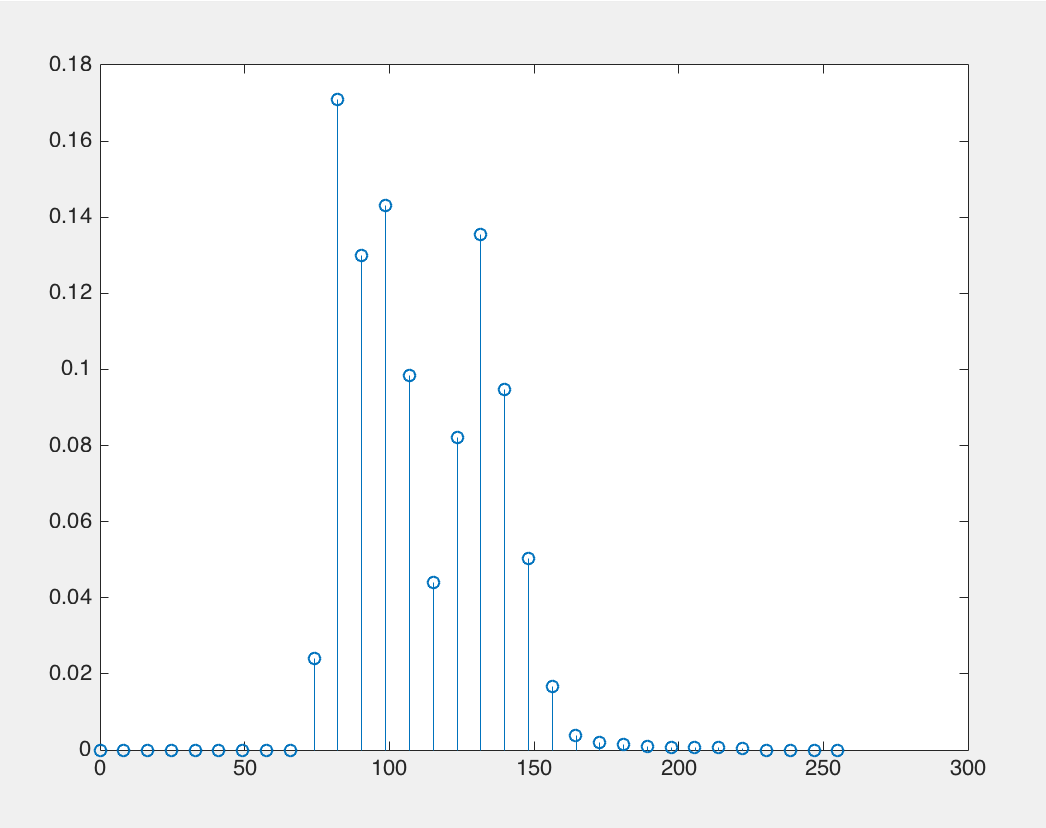#### 灰度的线性变换

$D_B = f(D_A) ＝f_AD_A +f_B$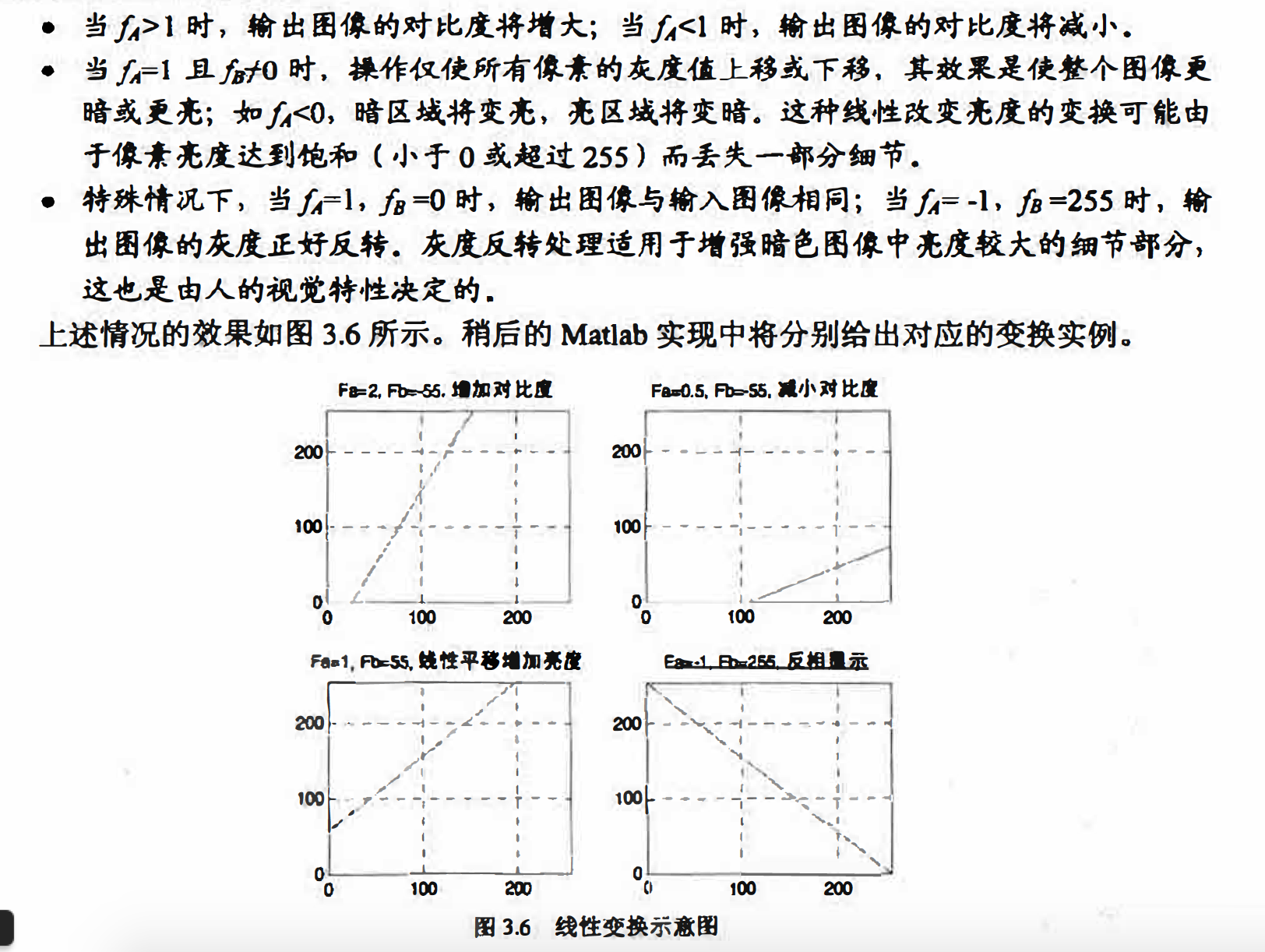matlab实现

I = imread('coins.png');		% 读入原图像

I = im2double(I);			% 转换数据类型为double
[M,N] = size(I);			% 计算图像面积

figure(1);				% 打开新窗口
imshow(I);				% 显示原图像
title('原图像');

figure(2);				% 打开新窗口
[H,x] = imhist(I, 64);		% 计算64个小区间的灰度直方图
stem(x, (H/M/N), '.');		% 显示原图像的直方图
title('原图像');

% 增加对比度
Fa = 2; Fb = -55;
O = Fa .* I + Fb/255;

figure(3);
subplot(2,2,1);
imshow(O);
title('Fa = 2 Fb = -55 增加对比度');

figure(4);
subplot(2,2,1);
[H,x] = imhist(O, 64);
stem(x, (H/M/N), '.');
title('Fa = 2 Fb = -55 增加对比度');

% 减小对比度
Fa = 0.5; Fb = -55;
O = Fa .* I + Fb/255;

figure(3);
subplot(2,2,2);
imshow(O);
title('Fa = 0.5 Fb = -55 减小对比度');

figure(4);
subplot(2,2,2);
[H,x] = imhist(O, 64);
stem(x, (H/M/N), '.');
title('Fa = 0.5 Fb = -55 减小对比度');

% 线性增加亮度
Fa = 1; Fb = 55;
O = Fa .* I + Fb/255;

figure(3);
subplot(2,2,3);
imshow(O);
title('Fa = 1 Fb = 55 线性平移增加亮度');

figure(4);
subplot(2,2,3);
[H,x] = imhist(O, 64);
stem(x, (H/M/N), '.');
title('Fa = 1 Fb = 55 线性平移增加亮度');

% 反相显示
Fa = -1; Fb = 255;
O = Fa .* I + Fb/255;

figure(3);
subplot(2,2,4);
imshow(O);
title('Fa = -1 Fb = 255 反相显示');

figure(4);
subplot(2,2,4);
[H,x] = imhist(O, 64);
stem(x, (H/M/N), '.');
title('Fa = -1 Fb = 255 反相显示');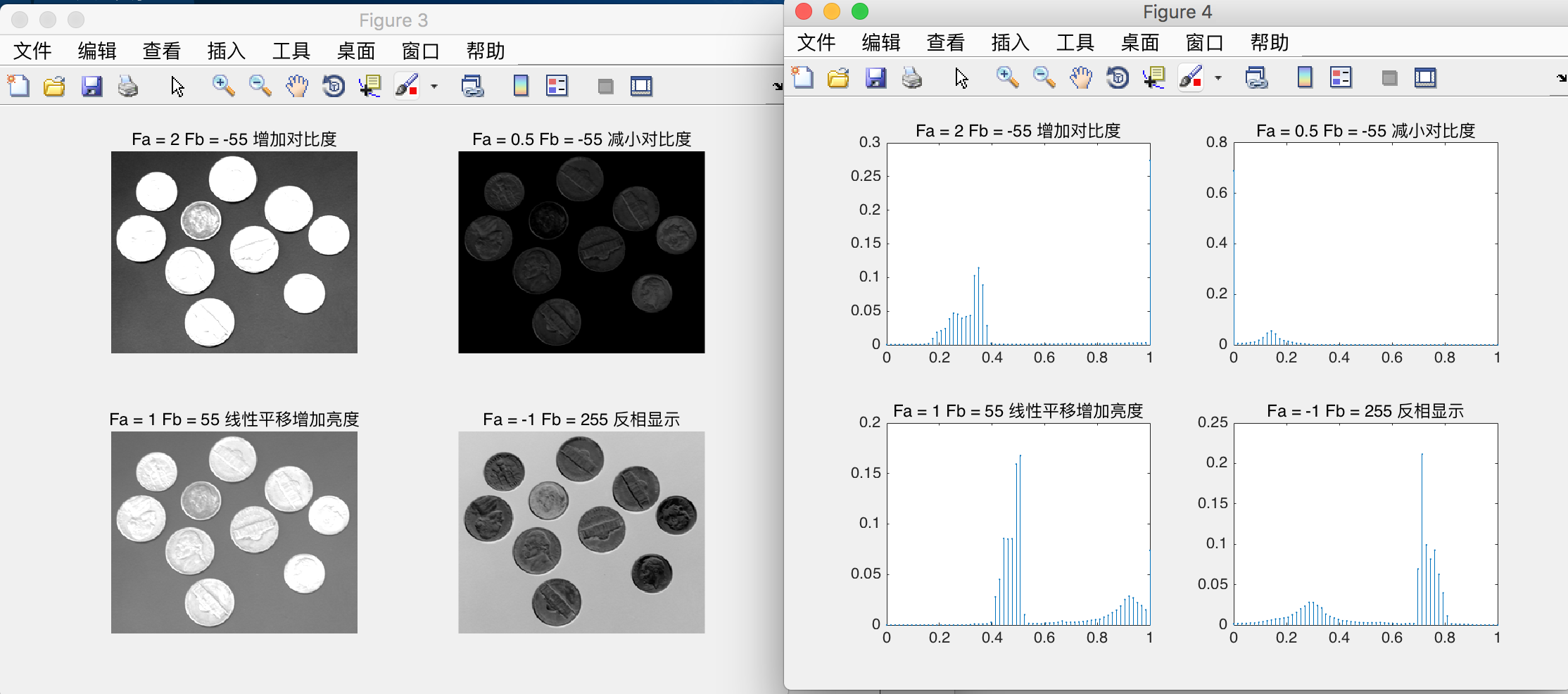#### 灰度对数变换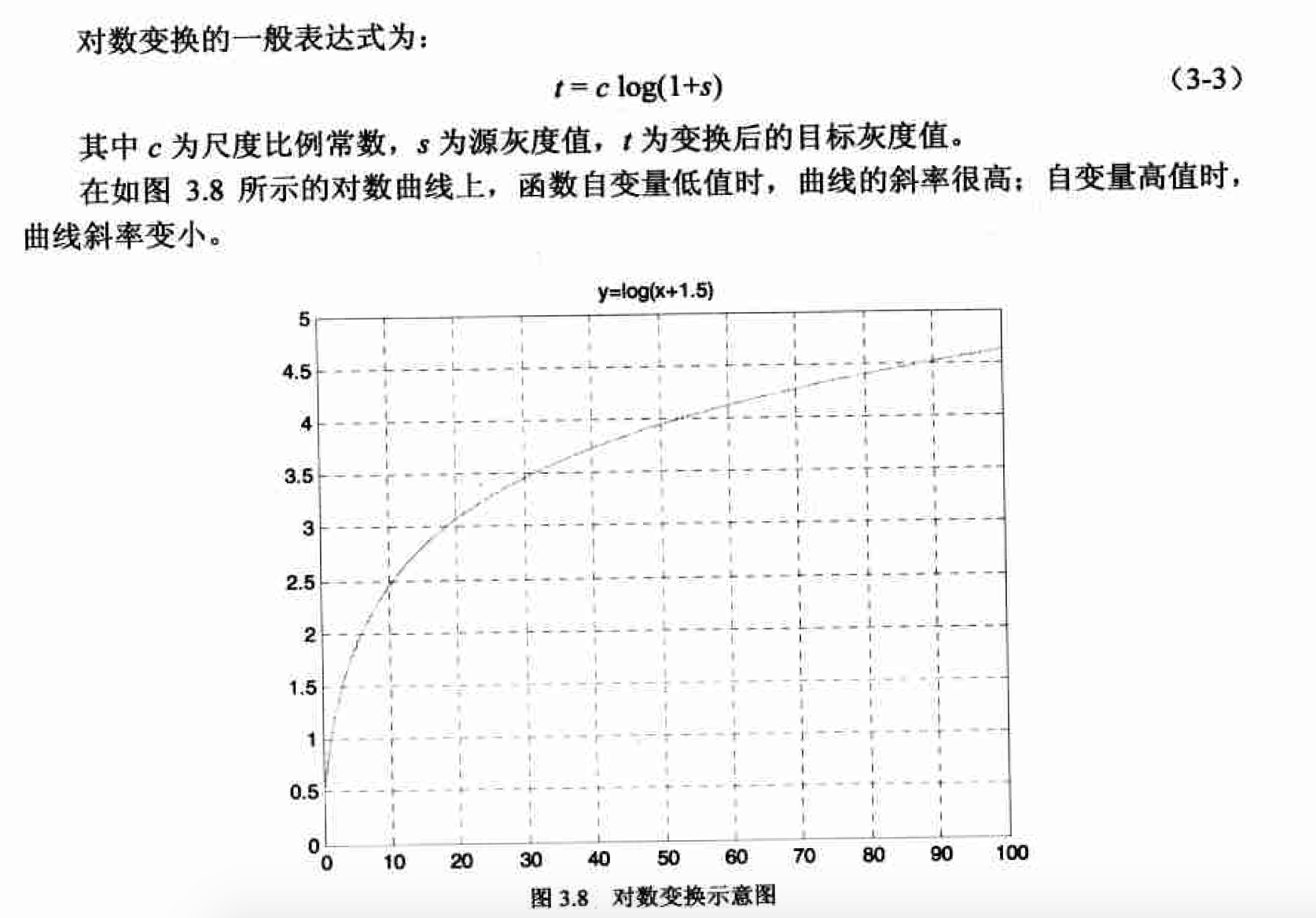matlab实现

I = imread('coins.png');
F = fft2(im2double(I));
F = fftshift(F);
F = abs(F);
T = log(F+1);

subplot(1,2,1);
imshow(F,[]);
title('未经变换的频谱');

subplot(1,2,2);
imshow(T,[]);
title('对数变换后');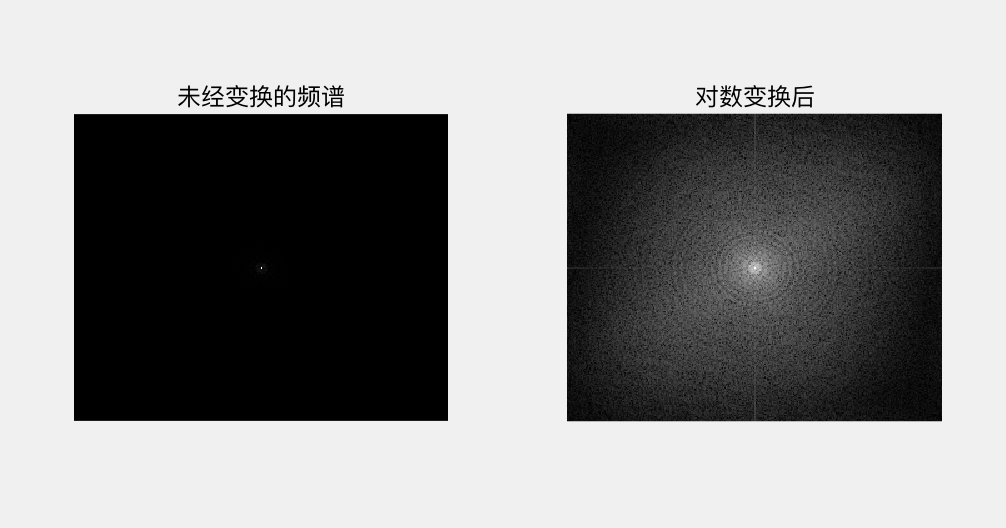#### 伽玛变换

$y=(x+esp)^{\gamma}$

• y>1时，图像的高灰度区域对比度得到增强；
• y<1时，图像的低灰皮区域对比度得到增强；
• y=1时，这一灰度变换是线性的，即不改变原图像．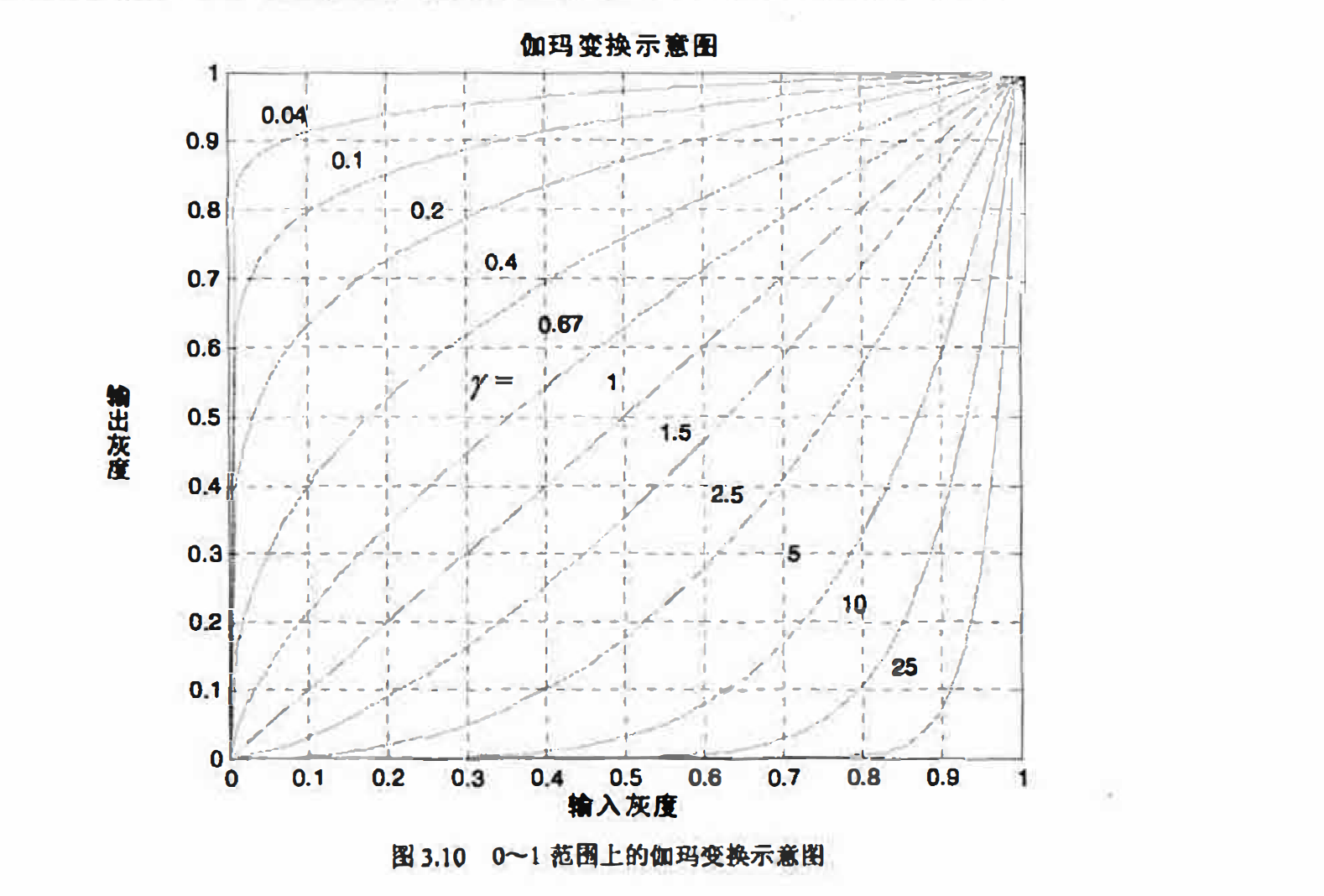matlab实现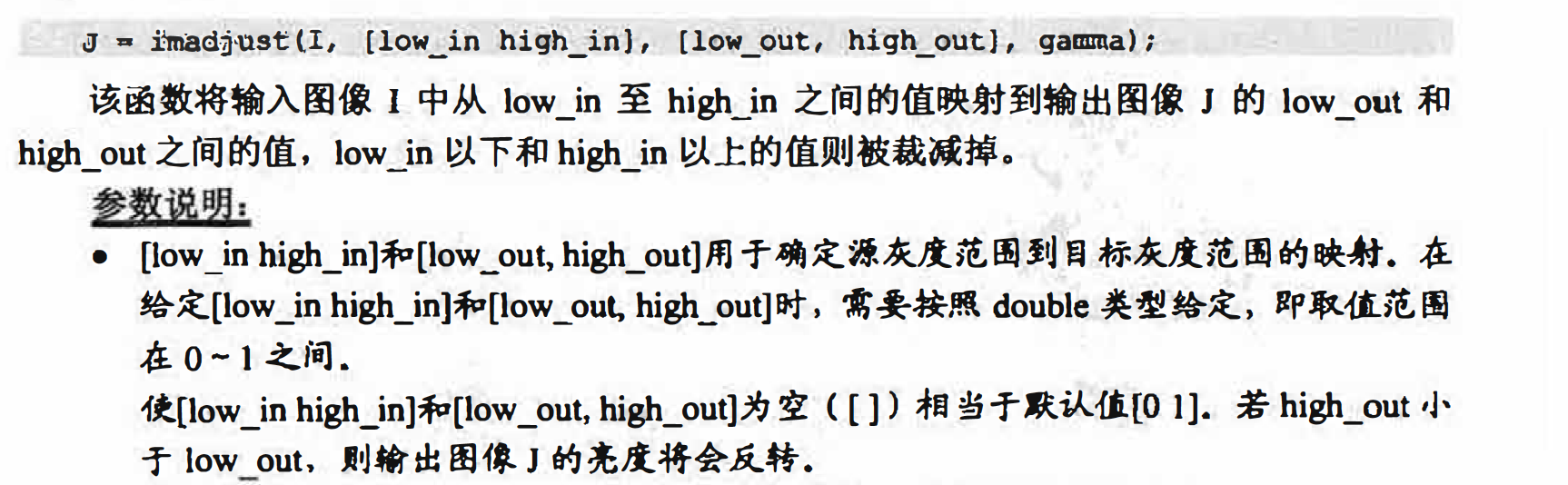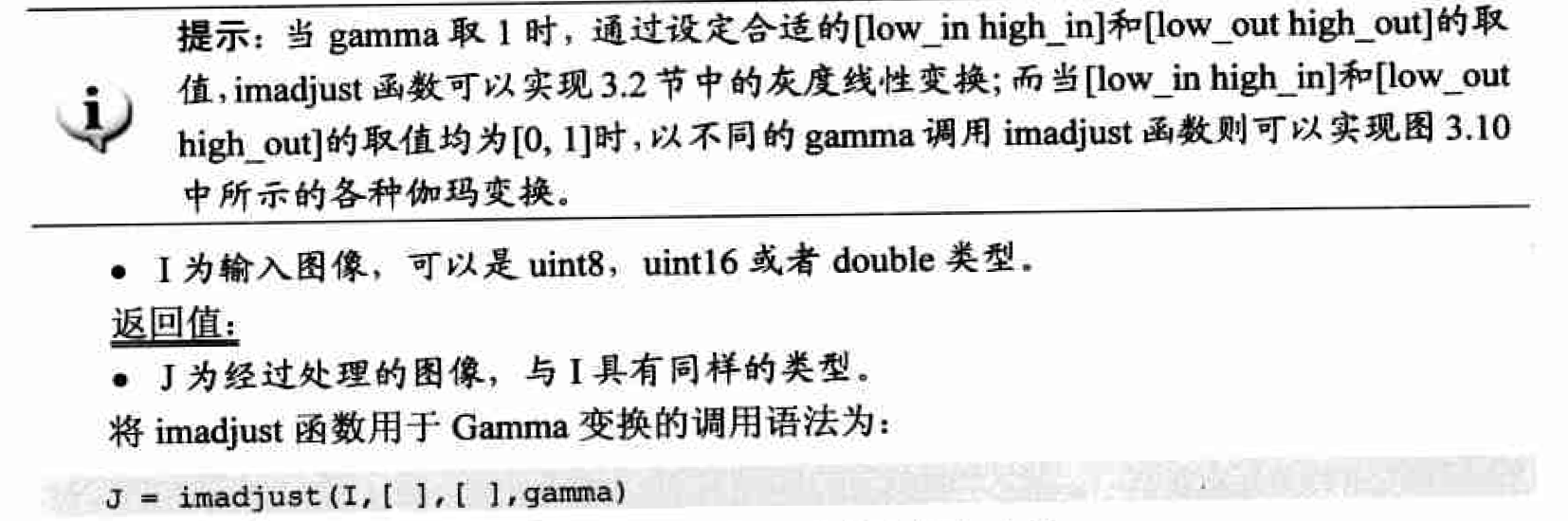I = imread('pout.tif');

subplot(1,3,1);
title('Gamma 0.75');

subplot(1,3,2);
title('Gamma 1');

subplot(1,3,3);
title('Gamma 1.5');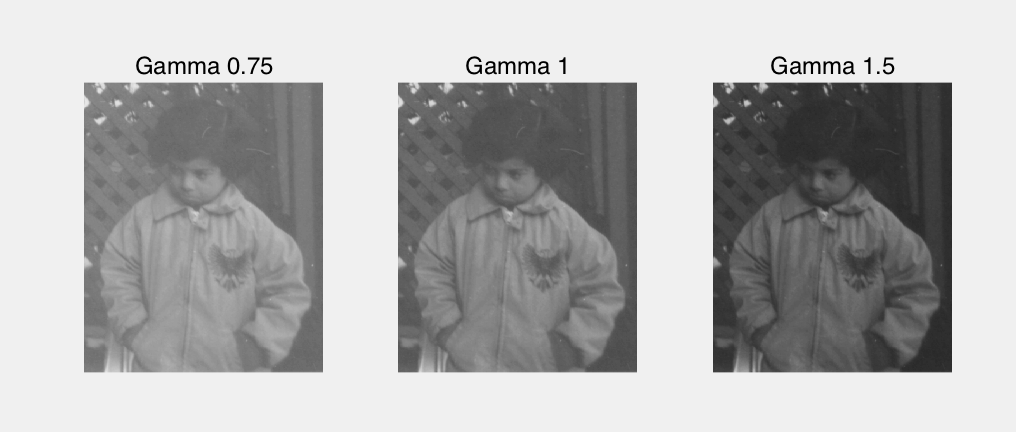I = imread('pout.tif');

subplot(1,3,1);
title('Gamma 0.75');

subplot(1,3,2);
title('Gamma 1');

subplot(1,3,3);
title('Gamma 1.5');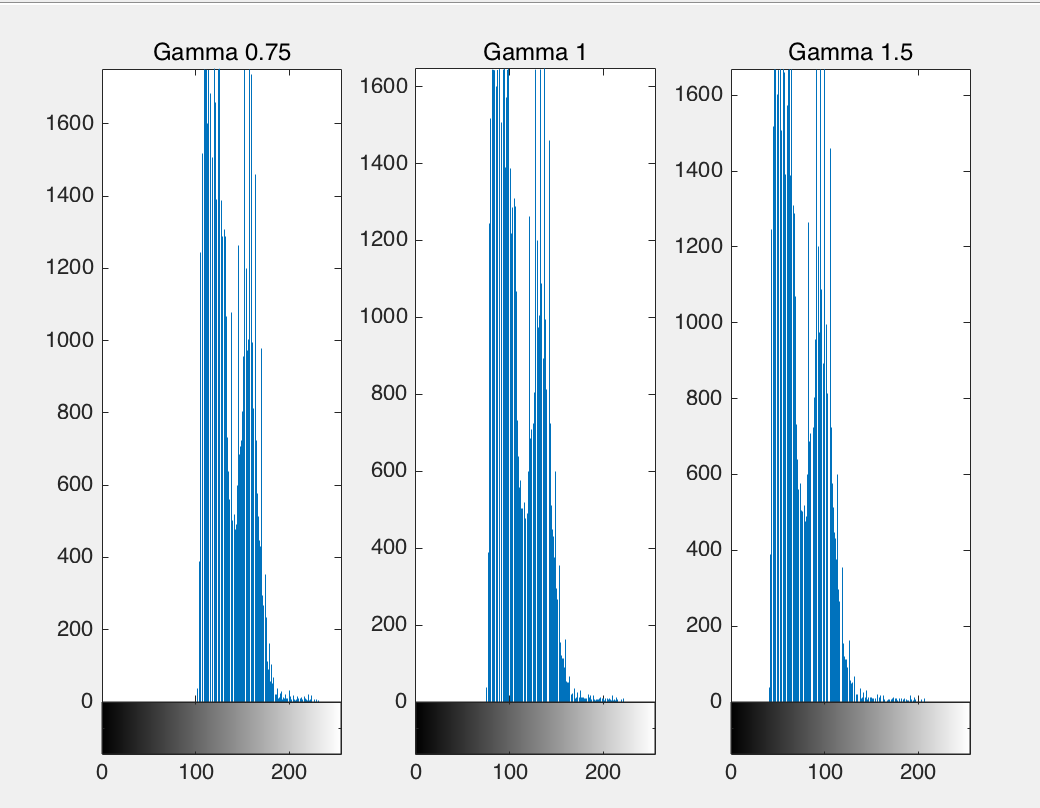注意图3.12中直方图非零区间位置的变化，以及这些变化给图像带来的影响。由于伽玛 变换并不是线性变换，所以它不仅可以改变图像的对比度，还能够增强细节，从而带来整体 图像效果的改善。

#### 灰度阙值变换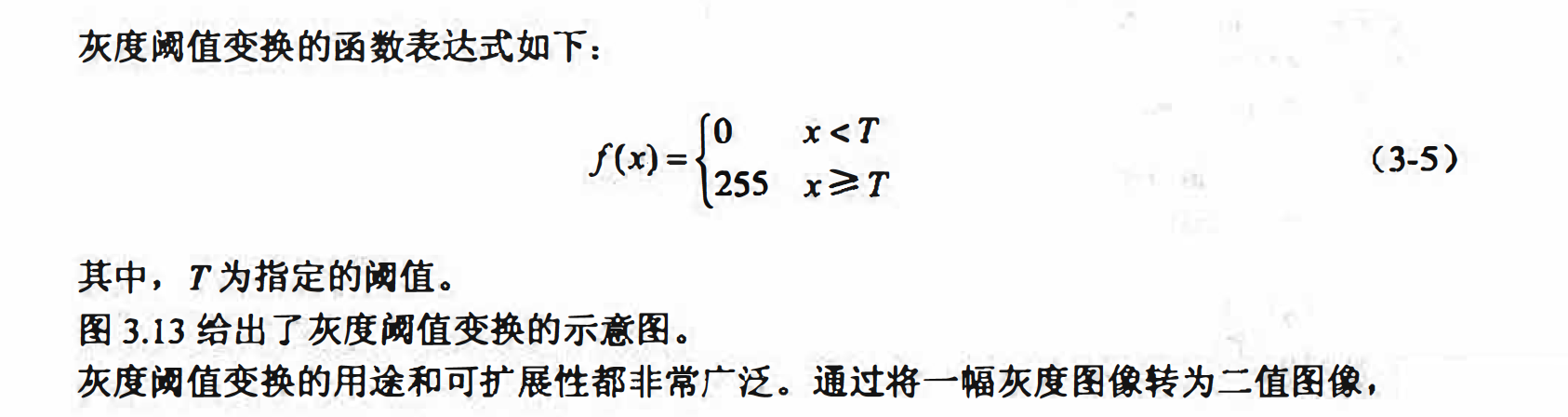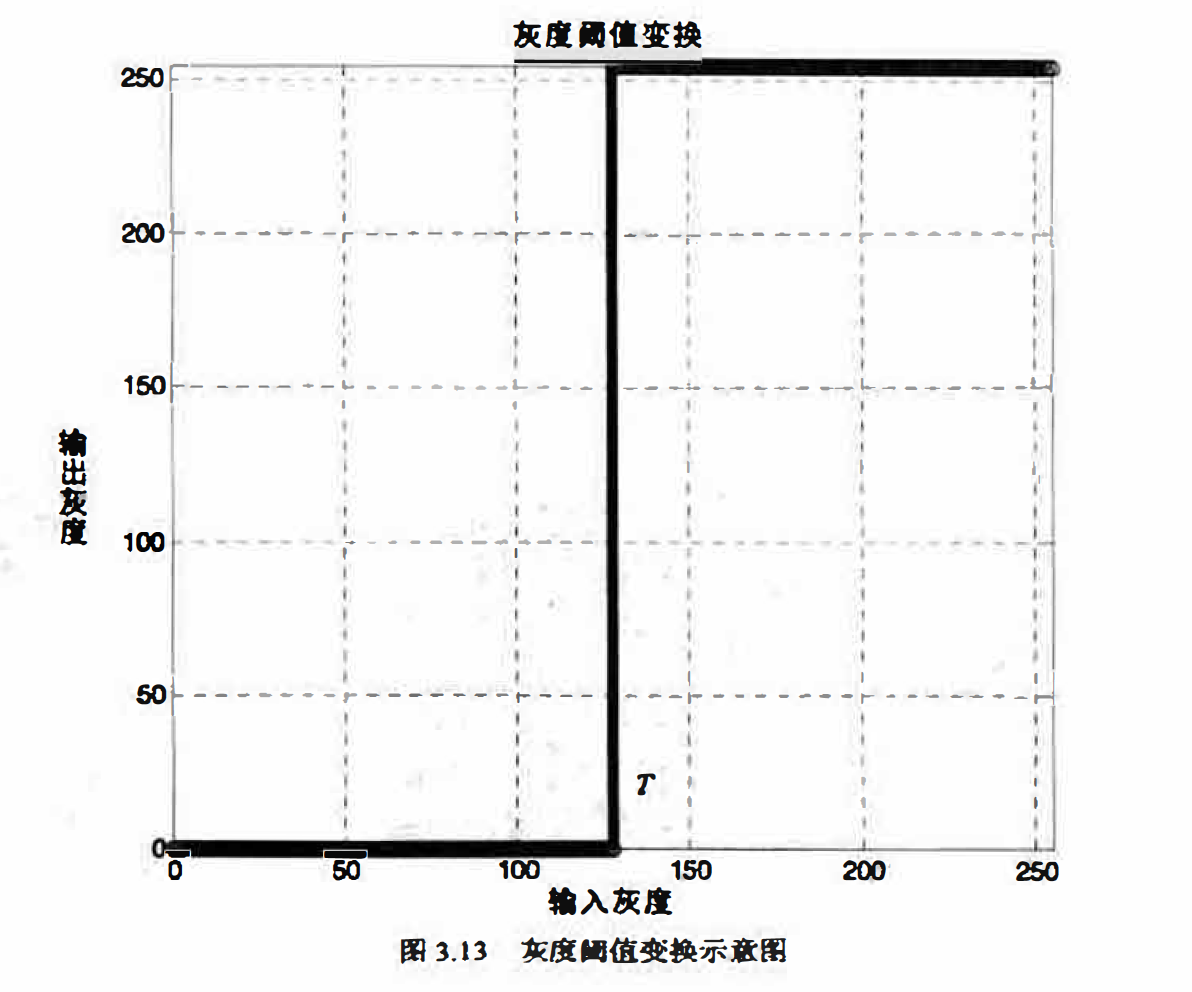matlab实现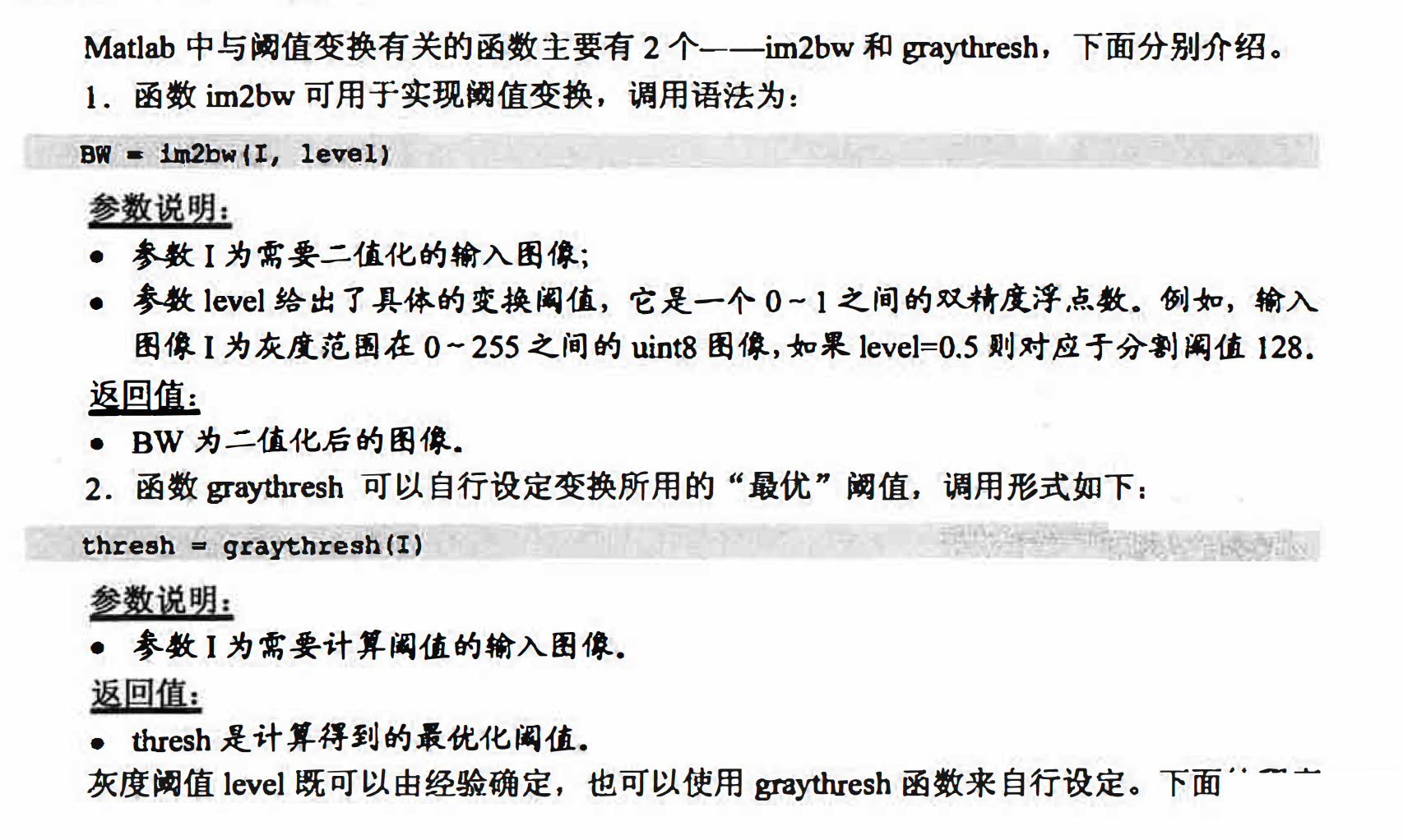I = imread('rice.png');
thresh = graythresh(I);
bw1=im2bw(I,thresh);
bw2=im2bw(I,130/255);
subplot(1,3,1);
imshow(I);
title('原图像');

subplot(1,3,2);
imshow(bw1);
title('自动选择阀值');

subplot(1,3,3);
imshow(bw2);
title('阀值130');